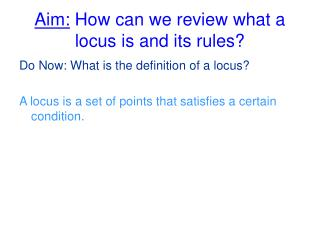DownloadDownload PresentationAim: How can we review what a locus is and its rules?

# Aim: How can we review what a locus is and its rules?

Télécharger la présentation## Aim: How can we review what a locus is and its rules?

- - - - - - - - - - - - - - - - - - - - - - - - - - - E N D - - - - - - - - - - - - - - - - - - - - - - - - - - -
##### Presentation Transcript

1. Aim: How can we review what a locus is and its rules? Do Now: What is the definition of a locus? A locus is a set of points that satisfies a certain condition.

2. There are 5 basic locus theorems: Theorem 1: The locus of points at a fixed distance, d, from point P is a circle with the given point P as its center and d as its radius. Theorem 2: The locus of points at a fixed distance, d, from a line l is a pair of parallel lines d distance from t and on either side of l. Theorem 3: The locus of points equidistance from two points, P and Q is the perpendicular bisector of the line segment determined by the two points.

3. Locus Theorem 4: The locus of points equidistance from two parallel lines, l1, 12, is a line parallel to both l1 and l2and midway between them Locus Theorem 5: The locus of points equidistant from two intersecting lines l1 and l2 is a pair of bisectors that bisect the angels formed by l1 and l2.

4. Steps to solving a locus problem: • Draw a diagram showing the given lines and points. • Read carefully to determine the needed condition(s). • Locate one point that satisfies the needed condition and plot it on your diagram. Locate several additional points that satisfy the condition and plot them as well. Plot enough points so that a pattern (a shape, a path) is starting to appear. • 4. Through these plotted points, draw a dotted line to indicate the locus (or path) of the points. • Describe in the words the geometric path that appears to be the locus. • If TWO conditions exist in your problem (a compound locus). Repeat steps 2-4 above for the second condition ON THE SAME DIAGRAM. Count the number of points where the two loci intersect. (Where do the dotted lines cross?)

5. A treasure is buried in your backyard. The picture below shows your backyard which contains a stump, a teepee, and a tree. The teepee is 8 feet from the stump and 18 feet from the tree. The treasure is equidistant from the teepee and the tree and also 6 feet from the stump. Locate all possible points of where the treasure is buried. The line represents the locus which is equidistant from the teepee and the tree the perpendicular bisector of the segment. The blue circle represents the locus which is 6 feet from the stump. These two loci intersect in two locations. The treasure could be buried at either “X” location. a) Draw the locus of points equidistance from the points (4.2) & (4.5) and write the equation for this locus. B) Draw the locus of points equidistance from the points (3,2) and (-4,2) and write the equation for this locus. c) Find the number of points that satisfy both conditions stated in a and b. Give the coordinates of each point found.

6. What is the equation of the locus of points 5 units away from the y-axis?

7. What is the equation of the locus of points equidistant from the linesy = -2 and y = 3?

8. True or False:  The locus of points equidistant from the sides of an angle is the angle bisector.

9. Two houses are 180 feet apart.  The underground electrical cable used to service the houses is to be placed such that the distance from any point on the cable to each home is always the same distance.  Describe where the cable should be placed.